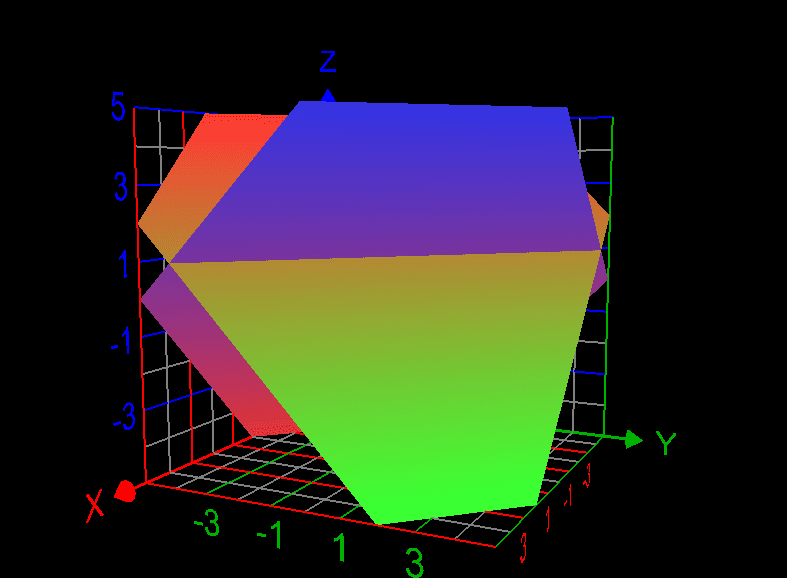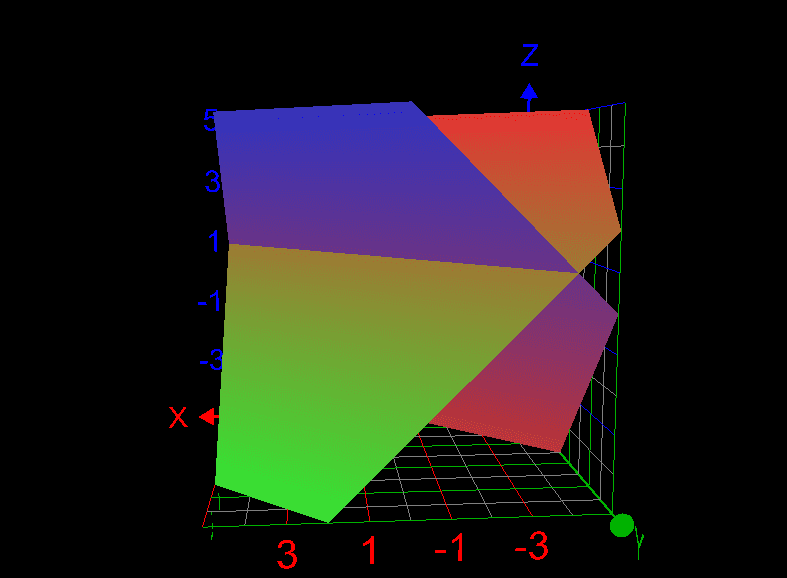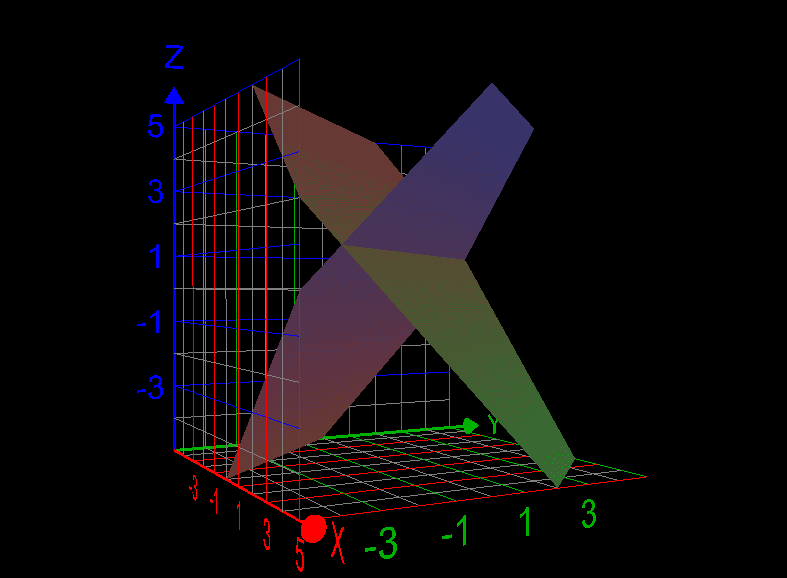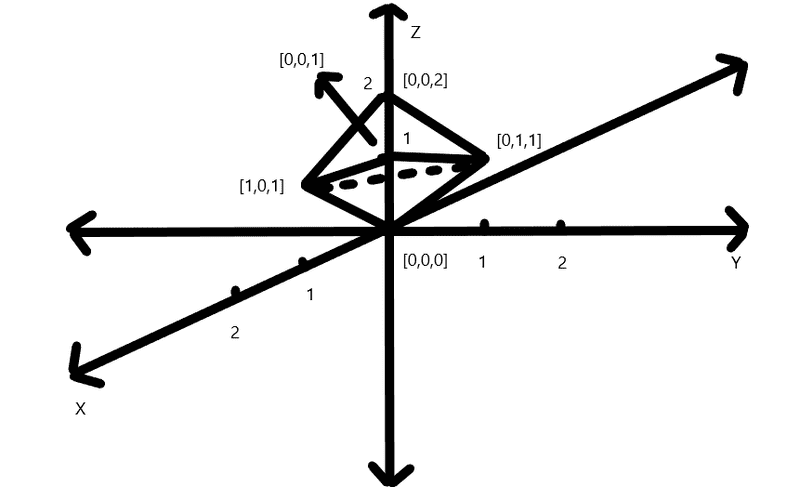# Finding the volume of the solid

WMDhamnekar
MHB
Homework Statement:
Find the volume V of the solid S bounded by the three coordinate planes, bounded above by the plane x + y + z = 2, and bounded below by the z = x + y.
Relevant Equations:
No equation
My attempt : ## \displaystyle\int_0^2 \displaystyle\int_0^{2-x} (2-2x -2y) dy dx = -\frac43 ## But it is wrong.

Mentor
Homework Statement:: Find the volume V of the solid S bounded by the three coordinate planes, bounded above by the plane x + y + z = 2, and bounded below by the z = x + y.
Relevant Equations:: No equation

My attempt : ## \displaystyle\int_0^2 \displaystyle\int_0^{2-x} (2-2x -2y) dy dx = -\frac43 ## But it is wrong.
Your first clue that you have done something wrong is that you got a negative number for the volume. Have you drawn a sketch of the solid? It looks like a pair of tetrahedrons, one on top of the other. At least one of your lower integration limits is wrong, as the base of the solid isn't in the x-y plane.

•WMDhamnekar
WMDhamnekar
MHB
Your first clue that you have done something wrong is that you got a negative number for the volume. Have you drawn a sketch of the solid? It looks like a pair of tetrahedrons, one on top of the other. At least one of your lower integration limits is wrong, as the base of the solid isn't in the x-y plane.
My graphing calculator sketched this problem as follows:Now, which volume, the author want to be computed by readers, viewers and/or students?

Mentor
The graphs from your calculator aren't very helpful. The sketch I drew on a piece of paper shows that the solid lies entirely in the first octant (the portion of space in which all three coordinates are nonnegative).

The vertices of the upper tetrahedron are at (0, 0, 2), (1, 0, 1), (0, 1, 1), and (0, 0, 1). Notice that the first three of these vertices lie on the plane z = 2 - x - y. The vertices of the lower tetrahedron are at (0, 0, 0), (1, 0, 1), (0, 1, 1), and (0, 0, 1). The first three of these vertices lie on the plane z = x + y.

•WMDhamnekar
WMDhamnekar
MHB
The graphs from your calculator aren't very helpful. The sketch I drew on a piece of paper shows that the solid lies entirely in the first octant (the portion of space in which all three coordinates are nonnegative).

The vertices of the upper tetrahedron are at (0, 0, 2), (1, 0, 1), (0, 1, 1), and (0, 0, 1). Notice that the first three of these vertices lie on the plane z = 2 - x - y. The vertices of the lower tetrahedron are at (0, 0, 0), (1, 0, 1), (0, 1, 1), and (0, 0, 1). The first three of these vertices lie on the plane z = x + y.
Hi,
Is the following sketch as per your vertices correct?Mentor
Hi,
Is the following sketch as per your vertices correct?View attachment 301525
Yes, that looks like what I did. I haven't worked the problem, but I think you'll need the equation of the line that goes from (1, 0, 1) over to (0, 1, 1). This should be x + y = 1, in the plane z = 1.

•WMDhamnekar
WMDhamnekar
MHB
Yes, that looks like what I did. I haven't worked the problem, but I think you'll need the equation of the line that goes from (1, 0, 1) over to (0, 1, 1). This should be x + y = 1, in the plane z = 1.
Sothe final answer to this question is ## \displaystyle\int_0^1\displaystyle\int_0^{1-x} (2-2x-2y) dy dx = \frac13 ##